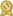# Ms. Pam S.

## specializes in Engr Instructor - 10+ years teaching Math, Physics, Statics... · female

"Engineers are problem solvers. Engineering is the merging of principles from math and science to create items of use. In engineering, I don’t teach new concepts, the theories have mostly already been covered in calculus and physics. My task is to get the students to think logically, work through problems using a systematic method, and help them develop good problem-solving habits.

There are many more...

"Engineers are problem solvers. Engineering is the merging of principles from math and science to create items of use. In engineering, I don’t teach new concepts, the theories have mostly already been covered in calculus and physics. My task is to get the students to think logically, work through problems using a systematic method, and help them develop good problem-solving habits.

There are many equations used in engineering. There are also many computations. I believe the process of problem solving is more important than memorizing the equations and “plugging and chugging” through the computations (we have the internet and computers for that!) I prefer to incorporate many examples in my tutoring. Often, I hear that the examples in the text are much easier than the homework problems, so my examples come from the problems at the end of the chapter. If we can work through a similar problem together, it gives the student confidence and persistence to work through the problems at home.

As an engineer, I know where the math can be used in a practical way. I can help with the computations of the math, but more importantly, let the student know how the math can be used and develop critical problem solving techniques to answer many different types of math problems.

I have taught the foundations of engineering for several years, Statics, Dynamics, Deformable Body Mechanics, Digital Electronics, Circuit Analysis and many others. Teachers often hear the same questions semester after semester, and we know where students are likely to have problems.

I have taught Solidworks for many years, and have attained the Accredited Educator status through Dassault Systemes (which produces Solidworks). My focus is on beginning and intermediate modeling, as well as sheet metal and drawings.

I would love to help you as you study math, physics or engineering. Having a tutor is so much more helpful than looking up answers on Chegg - we will work through the problems together! Feel free to contact me if you have" less...

# Details

 fee: \$65 (for 60 min) travel distance: 20 miles meeting type: either in-person or online

# Contact

 website: on file

# Education and Qualifications

 certified:Texas A&M University, Mechanical Engr

Texas A&M University, Masters

# Subjects Tutored

Algebra 1 - Algebra 2 - Physics - Prealgebra - Precalculus - Trigonometry - Elementary Math - Mechanical Engineering - Solidworks